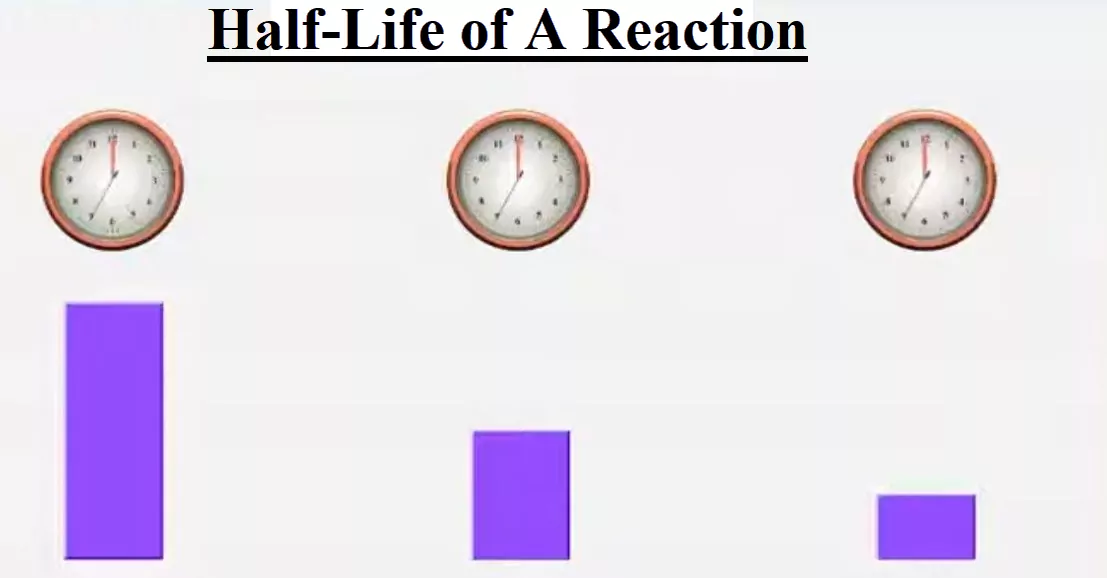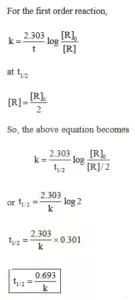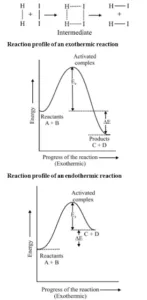# Half-Life of A ReactionHalf-Life of A Reaction

The half-life of a reaction (t1/2): It is the time in which the concentration of a reactant is reduced to one-half of its initial concentration. It is represented by t1/2.
(i) Half-Life of a Zero Order Reaction: For a zero-order reaction, the integrated rate law is:
k = [R0] – [R]
t
When t = t1/2, [R] = ½ [R0]
On substituting these values in the above equation,
k = [R0] – ½ [R0]
t1/2

 t1/2 = [R0]           2k

From the above relation, we can say Half-life of a zero-order reaction is directly proportional to the initial concentration of the reactants and inversely proportional to the rate constant.

Half-Life of A Reaction

(ii) Half-Life of a First Order Reaction: For a first-order reaction, the integrated rate law is:Half-Life of A Reaction

Rate of Reaction and Temperature: Most of the chemical reactions are accelerated by the increase in temperature. It has been found that for a chemical reaction when the temperature is increased by 10°, the rate of the reaction and the rate constant is nearly doubled.  The ratio of the rate constants of a reaction at two temperatures differing by 100 is called the temperature coefficient.
i.e., Temperature coefficient = Rate constant of the reaction at (T + 10)K
The rate constant of the reaction at T K
The temperature dependence of the rate of a chemical reaction can be accurately explained by the Arrhenius equation.  The equation is:
k = A e – Ea /RT ……………………………….. (1)

Where A is a constant called the Arrhenius parameter or the frequency factor or the pre-exponential factor. It is constant for a particular reaction.
R is the universal gas constant and Ea is activation energy measured in joules/mole (J mol –1).

Threshold Energy: The minimum energy which the colliding molecules must have in order that the collision between them may be effective is called threshold energy.

Activation Energy: The minimum extra amount of energy absorbed by the reactant molecules so that their energy becomes equal to the threshold value is called activation energy.
Threshold energy = Activation energy + Energy possessed by the reactants
Less is the activation energy, faster is the reaction. In order that the reactants may change into products, they have to cross an energy barrier (corresponding to threshold
energy). Reactant molecules absorb energy and form an intermediate called activated complex which immediately dissociates to form the products.
For Example:Half-Life of A Reaction